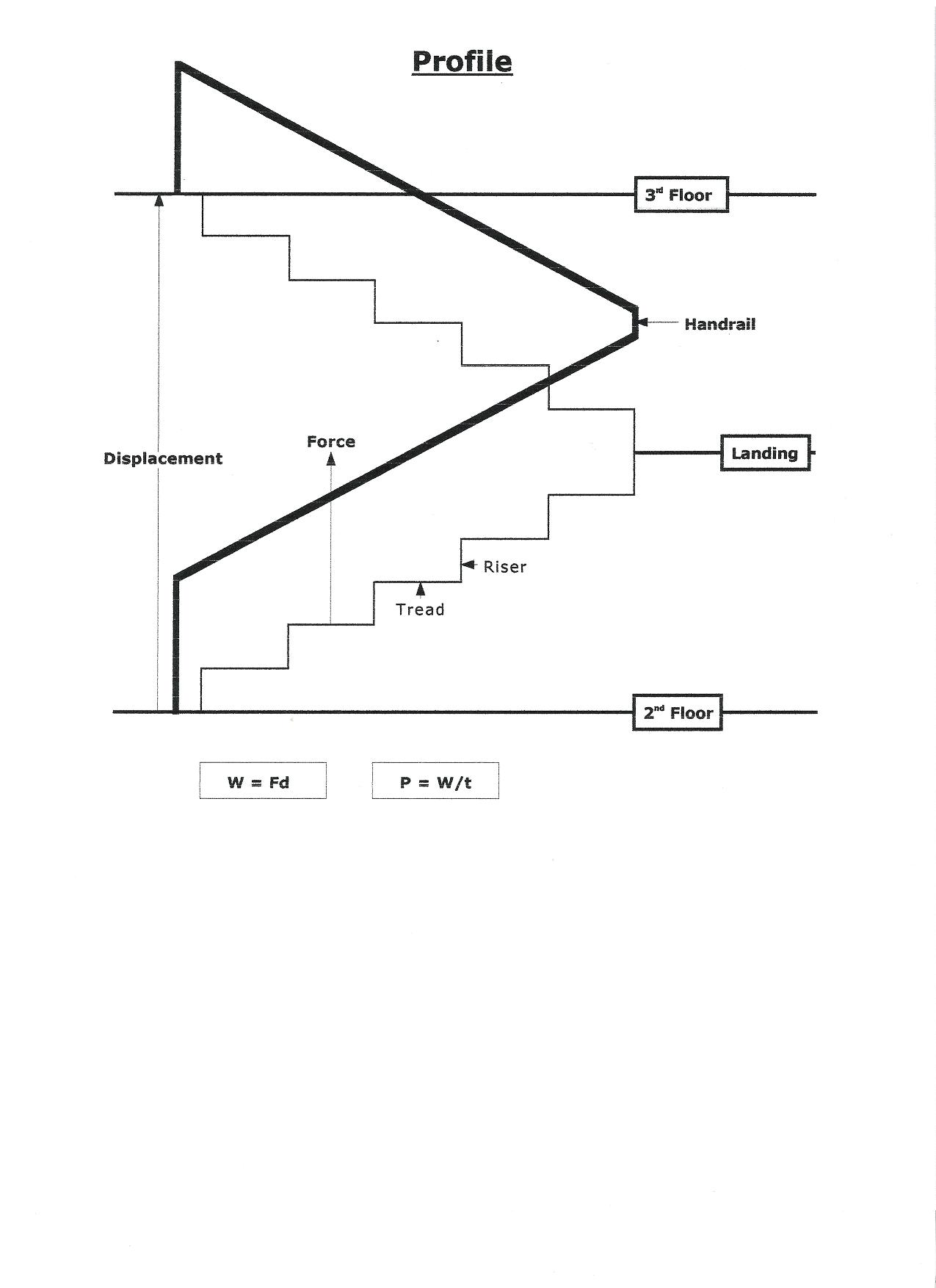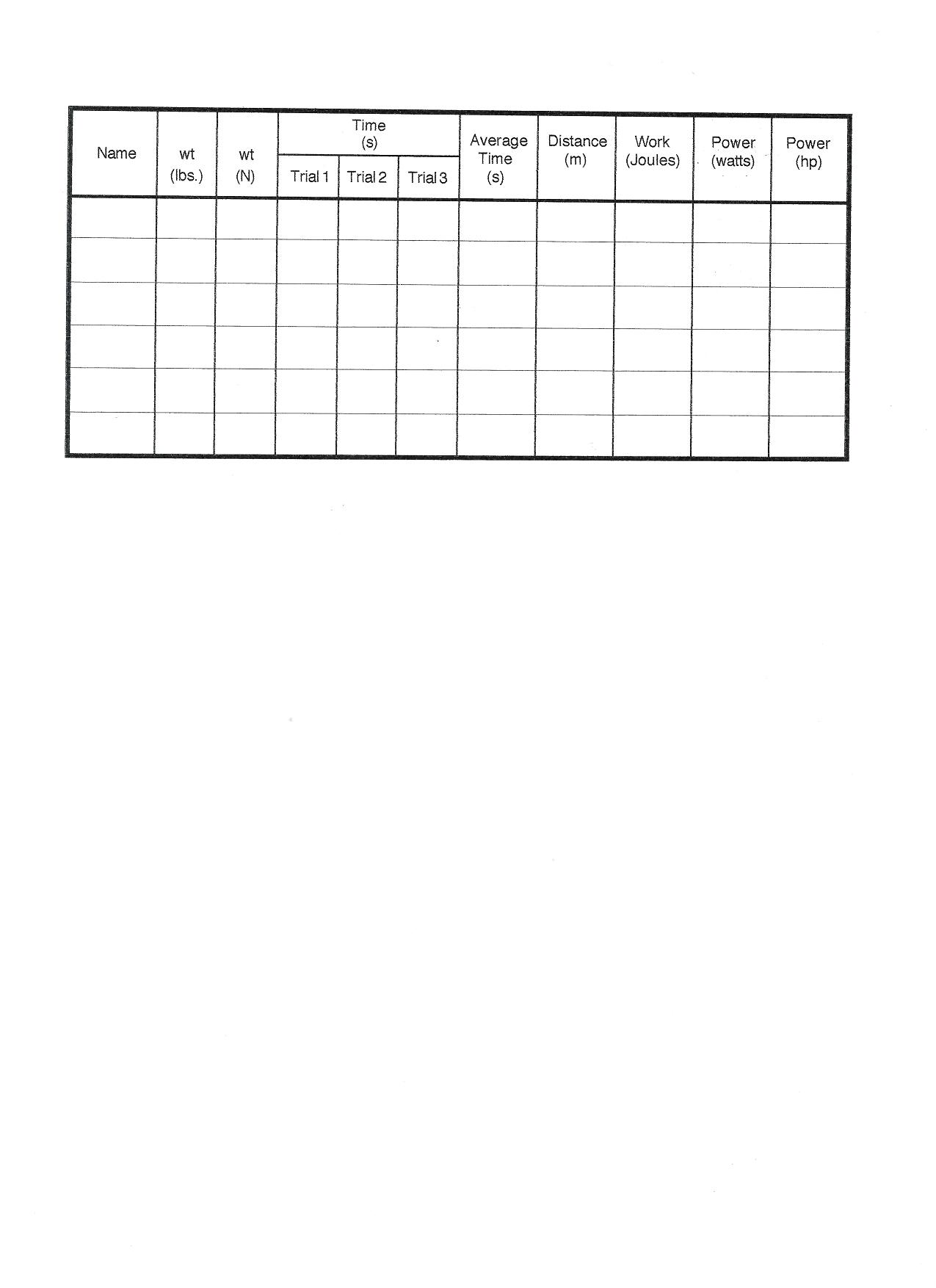Cycle II Regents Physics
John Dewey High School
Mr. Klimetz
Work and Power
What do Joules, Watts, and Horsepower All Share in Common?
Laboratory Exercise No. 5
How can we better understand the role of forces in doing work?
How can we better understand the physical and mathematical relationship between work and power?
How is power calculated?
How do we convert between SI and English units of power measurement?
IntroductionForce and Work. We are constantly using forces to move ourselves, vehicles, and bodies of all kinds from one place to another. When a force moves a body on which it acts in the direction of the force, we say it has done work. A mover lifting a box from the floor to a table or pushing a piano a few meters across the floor is exerting a force that is doing work. In each case, a force is actually moving the body in the direction in which the force is acting. The work done by a force is found by multiplying the force by the distance it has pushed the body in the direction of the force. The unit of work is obtained by simply combining the unit of force and the unit of distance. It is the newton-meter, which is also referred to as the joule (J). A newton-meter (or joule) is the work done by a force of one newton in moving an object a distance of one meter in the direction of the applied force.Power. We are often interested not only in how much work is done but also in how fast it is done. The rate at which work is done is called power. We determine the average power of a machine or device (or person) by dividing the work it does by the time it takes to complete it. The SI unit in which power is measured is the joule/second (J/s) or the watt (W). The power supplied by an engine, motor, etc., that does work at the rate of 1 joule per second is 1 watt. A watt is therefore the rate at which a force of 1 newton does work when it moves a body at the rate of 1 meter per second in the direction of the applied force. Since the watt is a relatively small unit of power, it is more often convenient to measure power in kilowatts (10exp3 watts). A kilowatt of power is equivalent to 1000 watts. A nonmetric unit of power commonly used in commerce and industry in the US is horsepower (hp). One horsepower is equivalent to 746 watts.People (and Horse) Power!  A simple way to measure the power output of a person is to measure the time it takes the person to run up a flight of stairs of known height. In this experiment you will compare your power output with that of your classmates (and, perhaps, of your somewhat out-of-shape but eminently good-natured instructor). You will then convert these units into horsepower units. When MKS power unit namesake James Watt invented his steam engine many years ago, he was asked how many horses his engine could replace. To find out, Watt constructed a rope-and-pulley device with which he could measure the time it took for a horse to lift various weights. Watt derived horsepower as the power a horse can produce steadily for a sustained period of time. How many horses would be necessary to replace Mr. K? (Don't answer that!)
Objectives
In this particular exercise you are going to
evaluate the role of forces in doing work,
explore the physical and mathematical relationship between work and power,
determine how power is calculated, and
convert between SI and English units of power measurement.
Equipment
Bathroom scale
Meter stick (or metric measuring tape)
Stopwatch
Procedure
1.   Locate a straight staircase at least 3.5 meters in vertical height and preferably with a handrailing.  There should also be a clear area of at least two meters at both the top and bottom of the stairs. Measure the height of one single riser (step face) and multiply this by the total number of steps and record. This is your total displacement over which work will be done.
2.  Use the bathroom scale to measure the weight of each person in your group. Since the bathroom scale is calibrated in English units you must convert the bathroom scale reading reading to metric units (N). Note that 1 pound equals 4.45 N.
3.  Set the stopwatch chronometer function to zero. Ask the first member to then run up the stairs between adjacent floors (first floor to second floor or second floor to third floor) as fast as possible. (Remind all your fellow students before beginning their run to grasp the handrailing for safety as well as for added power during their ascent.) Commence timing when both of each runner's feet have left the floor upon which they began their trip and stop the timing when both feet are on the top floor of the landing at the end of their trip. Record the time in seconds.
4.  Repeat the procedure for each group member. Record all data. Some team members may wish to repeat their trials to improve their travel times.
5.  Perform all calculations and conversions.
6.  Answer all questions at the end of the exercise.
Data Table
Name

__________

__________

__________

__________

__________

__________
wt (lbs)
wt (N)
Trial 1
Trial 2
Trial 3
Avg.
Time
(s)
Time
(s)
Distance
Run
(m)
Work
Done
(J)
Power
Generated
(W)
Power
Generated
(hp)_____________________________________________________________________________
_____________________________________________________________________________
_____________________________________________________________________________
_____________________________________________________________________________
_____________________________________________________________________________
_____________________________________________________________________________
Calculations
1.  Use the following equation to compute the power output (in watts) of each group member listed in the data table:
P = (F x d)/t
where P is power (watts), F is applied force (newtons), d is displacement traveled in the direction of the applied force (meters) and t is time (seconds). Record where indicated.
2.  Convert the wattage of each participant into horsepower equivalents and record.
Questions
1.  What is the relationship between the horsepower of the participants in this experiment and James Watt's definition of the horsepower? In other words, compare the calculated horsepower readings of each group member.
2.  List potential sources of error in this experiment.
3.  How does this experiment illustrate the relationship between force, displacement, and time in the power equation?
4. What should a graph of force versus distance resemble if we were to run up different flights of stairs of different heights? Sketch the presumed relationship, assuming that we were running at top speed and we never ran out of energy. To what is the area beneath the plotted line equivalent? (Think!)